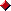Documentation

The Java™ Tutorials
Trail: Learning the Java Language
Lesson: Classes and Objects

# Answers to Questions and Exercises: Classes

## Questions

1. Consider the following class:

```public class IdentifyMyParts {
public static int x = 7;
public int y = 3;
}
```
1. Question: What are the class variables?

2. Question: What are the instance variables?

3. Question: What is the output from the following code:

```IdentifyMyParts a = new IdentifyMyParts();
IdentifyMyParts b = new IdentifyMyParts();
a.y = 5;
b.y = 6;
a.x = 1;
b.x = 2;
System.out.println("a.y = " + a.y);
System.out.println("b.y = " + b.y);
System.out.println("a.x = " + a.x);
System.out.println("b.x = " + b.x);
System.out.println("IdentifyMyParts.x = " + IdentifyMyParts.x);
```

Answer: Here is the output:

``` a.y = 5
b.y = 6
a.x = 2
b.x = 2
IdentifyMyParts.x = 2
```

Because `x` is defined as a `public static int` in the class `IdentifyMyParts`, every reference to `x` will have the value that was last assigned because `x` is a static variable (and therefore a class variable) shared across all instances of the class. That is, there is only one `x`: when the value of `x` changes in any instance it affects the value of `x` for all instances of `IdentifyMyParts`.

This is covered in the Class Variables section of Understanding Instance and Class Members.

## Exercises

1. Exercise: Write a class whose instances represent a single playing card from a deck of cards. Playing cards have two distinguishing properties: rank and suit. Be sure to keep your solution as you will be asked to rewrite it in Enum Types.

Answer: `Card.java`.

2. Exercise: Write a class whose instances represents a full deck of cards. You should also keep this solution.

Answer: See `Deck.java`.

3. Exercise: Write a small program to test your deck and card classes. The program can be as simple as creating a deck of cards and displaying its cards.

Answer: See `DisplayDeck.java`.

Previous page: Questions and Exercises: Classes